In :
from pylab import *
import numpy as np
import matplotlib.pyplot as plt
import matplotlib.cbook as cbook
import time
from scipy.misc import imresize
import matplotlib.image as mpimg
from scipy.ndimage import filters
import urllib
from numpy import random

import os

import torch

t = int(0)
random.seed(t)

from urllib.request import urlopen

heartshape_file = "https://raw.githubusercontent.com/guerzh/deeplearningvalentine/master/v.png"
heart = imresize(heart, .3)
heart = sum(heart, 2).astype(float)
heart /= float(max(heart.flatten()))
imshow(heart, cmap = cm.gray)
figure(1)
show()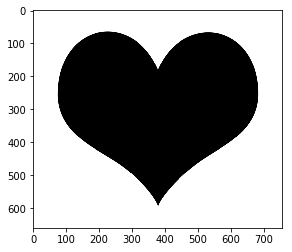Now, let's generate the dataset:

In :
def generate_imset(im, set_size):
x1 = np.random.randint(0, im.shape-1, set_size)
x2 = np.random.randint(0, im.shape-1, set_size)

x = vstack((x2, x1)).T

y_bool = (im[im.shape-1-x1, x2] < .5)
y1 = y_bool.astype(float)
y2 = (1 - y_bool).astype(float)

y = vstack((y1, y2)).T

return x, y

In :
train_size = 1250
test_size = 1000
train_set_x, train_set_y = generate_imset(heart, train_size)
test_set_x, test_set_y = generate_imset(heart, test_size)

figure(2)
scatter(train_set_x[train_set_y[:,0]==1.0,0], train_set_x[train_set_y[:,0]==1.0,1], color="r")
scatter(train_set_x[train_set_y[:,0]==0.0,0], train_set_x[train_set_y[:,0]==0.0,1], color="b")

Out:
<matplotlib.collections.PathCollection at 0x7f021684a5f8>
In :
show()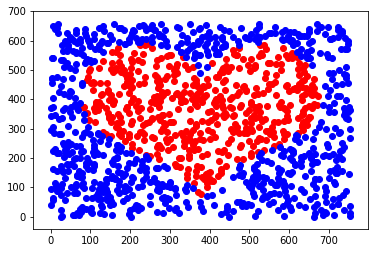We now have a dataset: the red points are 1's and the blue points are 0's. The inputs are just the coordinates.

A bit of housekeeping: let's write a function that returns minibatches:

In :
def get_batch(set_x, set_y, N):
idx = array(random.permutation(set_x.shape)[:N])
batch_xs = set_x[idx, :]
batch_ys = set_y[idx, :]

return batch_xs, batch_ys


Now let's define our network

In :
dim_x = 2
dim_h = 40
dim_out = 2

model = torch.nn.Sequential(
torch.nn.Linear(dim_x, dim_h),
torch.nn.ReLU(),
torch.nn.Linear(dim_h, dim_out),
)


PyTorch's default initialization is very far away from what we want

In :
model.weight.data = model.weight.data/10000.0
model.weight.data = model.weight.data/10000.0


More housekeeping:

In :
loss_fn = torch.nn.CrossEntropyLoss()

learning_rate = 1e-3

heart_out = 0


Now let's train, while visualizing the prediction for each coordinate every T timesteps

In :
T = 400

In :
try:
os.mkdir("new")
except:
print("Warning: Folder named new not created")

for t in range(5000):
batch_xs, batch_ys = get_batch(train_set_x, train_set_y, 1000)
x = Variable(torch.from_numpy(batch_xs.astype(np.float32)))
y_classes = Variable(torch.from_numpy(np.argmax(batch_ys, 1)))

y_pred = model(x)
loss = loss_fn(y_pred, y_classes)
loss.backward()
optimizer.step()

if t % T == 0:

class_performance = np.mean( (y_pred[:,1].data.numpy()>0) == y_classes.data.numpy())
print("loss=", loss)
print("classification performance", class_performance)

x1, x2 = np.meshgrid(np.arange(0, heart.shape).astype(np.float32), np.arange(0,heart.shape).astype(np.float32))

all_x =  Variable(torch.from_numpy(vstack((x2.flatten(), x1.flatten())).T))
all_y = torch.nn.Softmax()(model(all_x))

all_y0 = all_y[:, 0].data.numpy()
all_y0 = all_y0.reshape((heart.shape, heart.shape)).T

fig = figure(heart_out)
axis("off")

scatter(train_set_x[train_set_y[:,0]==1.0,0], train_set_x[train_set_y[:,0]==1.0,1], color="r")
scatter(train_set_x[train_set_y[:,0]==0.0,0], train_set_x[train_set_y[:,0]==0.0,1], color="b")

imshow(all_y0, origin="lower", cmap = plt.cm.jet)

fig.savefig("new/im%.4d.png" % heart_out)
plt.show()

heart_out += 1


Warning: Folder named new not created
loss= Variable containing:
0.6955
[torch.FloatTensor of size 1]

classification performance 0.581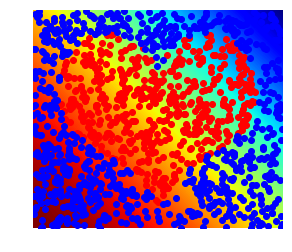loss= Variable containing:
0.4435
[torch.FloatTensor of size 1]

classification performance 0.797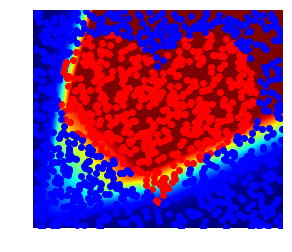loss= Variable containing:
0.4140
[torch.FloatTensor of size 1]

classification performance 0.794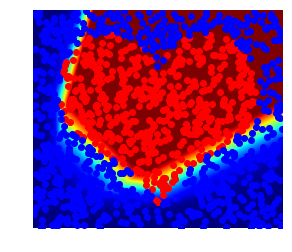loss= Variable containing:
0.3971
[torch.FloatTensor of size 1]

classification performance 0.803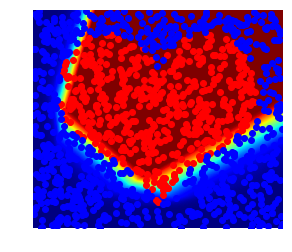loss= Variable containing:
0.3319
[torch.FloatTensor of size 1]

classification performance 0.835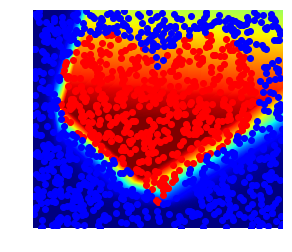loss= Variable containing:
0.2248
[torch.FloatTensor of size 1]

classification performance 0.94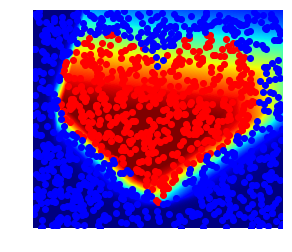loss= Variable containing:
0.1866
[torch.FloatTensor of size 1]

classification performance 0.946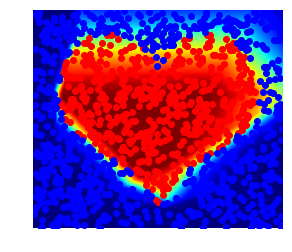loss= Variable containing:
0.1581
[torch.FloatTensor of size 1]

classification performance 0.955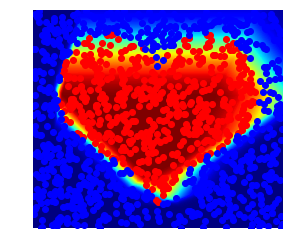loss= Variable containing:
0.1501
[torch.FloatTensor of size 1]

classification performance 0.962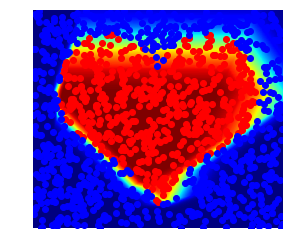loss= Variable containing:
0.1390
[torch.FloatTensor of size 1]

classification performance 0.952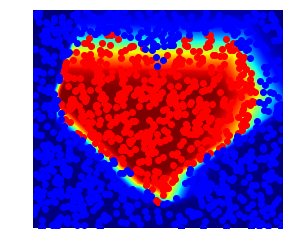loss= Variable containing:
0.1321
[torch.FloatTensor of size 1]

classification performance 0.951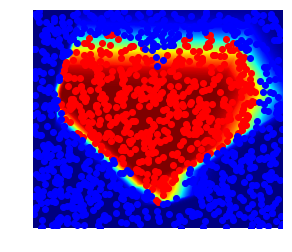loss= Variable containing:
0.1189
[torch.FloatTensor of size 1]

classification performance 0.966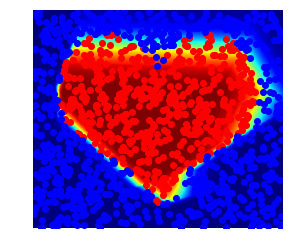loss= Variable containing:
0.1238
[torch.FloatTensor of size 1]

classification performance 0.949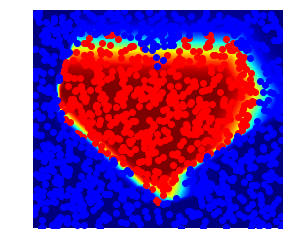In [ ]: